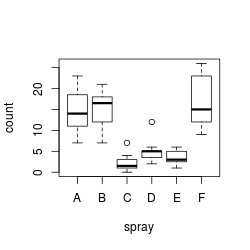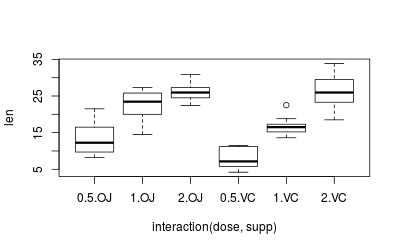## Problem

You want test samples to see for homogeneity of variance (homoscedasticity) – or more accurately. Many statistical tests assume that the populations are homoscedastic.

## Solution

There are many ways of testing data for homogeneity of variance. Three methods are shown here.

• Bartlett’s test - If the data is normally distributed, this is the best test to use. It is sensitive to data which is not non-normally distribution; it is more likely to return a “false positive” when the data is non-normal.
• Levene’s test - this is more robust to departures from normality than Bartlett’s test. It is in the `car` package.
• Fligner-Killeen test - this is a non-parametric test which is very robust against departures from normality.

For all these tests, the null hypothesis is that all populations variances are equal; the alternative hypothesis is that at least two of them differ.

### Sample data

The examples here will use the `InsectSprays` and `ToothGrowth` data sets. The `InsectSprays` data set has one independent variable, while the `ToothGrowth` data set has two independent variables.

``````head(InsectSprays)
#>   count spray
#> 1    10     A
#> 2     7     A
#> 3    20     A
#> 4    14     A
#> 5    14     A
#> 6    12     A

tg      <- ToothGrowth
tg\$dose <- factor(tg\$dose) # Treat this column as a factor, not numeric
#>    len supp dose
#> 1  4.2   VC  0.5
#> 2 11.5   VC  0.5
#> 3  7.3   VC  0.5
#> 4  5.8   VC  0.5
#> 5  6.4   VC  0.5
#> 6 10.0   VC  0.5
``````

Quick boxplots of these data sets:

``````plot(count ~ spray, data = InsectSprays)
````````````plot(len ~ interaction(dose,supp), data=ToothGrowth)
``````On a first glance, it appears that both data sets are heteroscedastic, but this needs to be properly tested, which we’ll do below.

### Bartlett’s test

With one independent variable:

``````bartlett.test(count ~ spray, data=InsectSprays)
#>
#> 	Bartlett test of homogeneity of variances
#>
#> data:  count by spray
#> Bartlett's K-squared = 25.96, df = 5, p-value = 9.085e-05

# Same effect, but with two vectors, instead of two columns from a data frame
# bartlett.test(InsectSprays\$count ~ InsectSprays\$spray)
``````

With multiple independent variables, the `interaction()` function must be used to collapse the IV’s into a single variable with all combinations of the factors. If it is not used, then the will be the wrong degrees of freedom, and the p-value will be wrong.

``````bartlett.test(len ~ interaction(supp,dose), data=ToothGrowth)
#>
#> 	Bartlett test of homogeneity of variances
#>
#> data:  len by interaction(supp, dose)
#> Bartlett's K-squared = 6.9273, df = 5, p-value = 0.2261

# The above gives the same result as testing len vs. dose alone, without supp
bartlett.test(len ~ dose, data=ToothGrowth)
#>
#> 	Bartlett test of homogeneity of variances
#>
#> data:  len by dose
#> Bartlett's K-squared = 0.66547, df = 2, p-value = 0.717
``````

### Levene’s test

The `leveneTest` function is part of the `car` package.

With one independent variable:

``````library(car)

leveneTest(count ~ spray, data=InsectSprays)
#> Levene's Test for Homogeneity of Variance (center = median)
#>       Df F value   Pr(>F)
#> group  5  3.8214 0.004223 **
#>       66
#> ---
#> Signif. codes:  0 '***' 0.001 '**' 0.01 '*' 0.05 '.' 0.1 ' ' 1
``````

With two independent variables. Note that the `interaction` function is not needed, as it is for the other two tests.

``````leveneTest(len ~ supp*dose, data=tg)
#> Levene's Test for Homogeneity of Variance (center = median)
#>       Df F value Pr(>F)
#> group  5  1.7086 0.1484
#>       54
``````

### Fligner-Killeen test

With one independent variable:

``````fligner.test(count ~ spray, data=InsectSprays)
#>
#> 	Fligner-Killeen test of homogeneity of variances
#>
#> data:  count by spray
#> Fligner-Killeen:med chi-squared = 14.483, df = 5, p-value = 0.01282

# Same effect, but with two vectors, instead of two columns from a data frame
# fligner.test(InsectSprays\$count ~ InsectSprays\$spray)
``````

The `fligner.test` function has the same quirks as `bartlett.test` when working with multiple IV’s. With multiple independent variables, the `interaction()` function must be used.

``````fligner.test(len ~ interaction(supp,dose), data=ToothGrowth)
#>
#> 	Fligner-Killeen test of homogeneity of variances
#>
#> data:  len by interaction(supp, dose)
#> Fligner-Killeen:med chi-squared = 7.7488, df = 5, p-value = 0.1706

# The above gives the same result as testing len vs. dose alone, without supp
fligner.test(len ~ dose, data=ToothGrowth)
#>
#> 	Fligner-Killeen test of homogeneity of variances
#>
#> data:  len by dose
#> Fligner-Killeen:med chi-squared = 1.3879, df = 2, p-value = 0.4996
``````